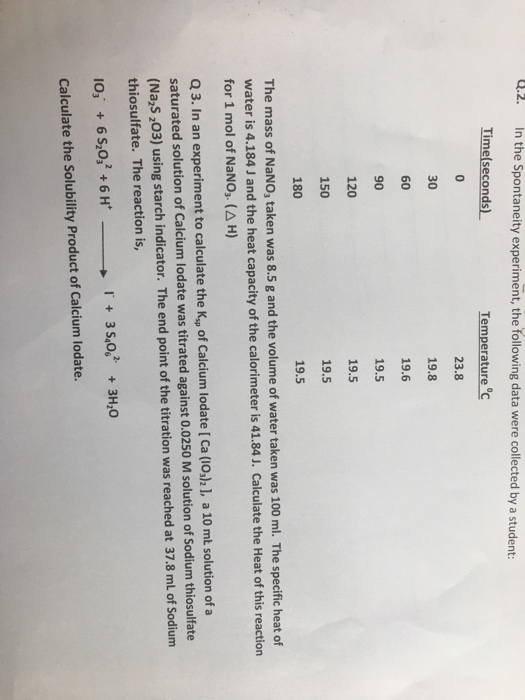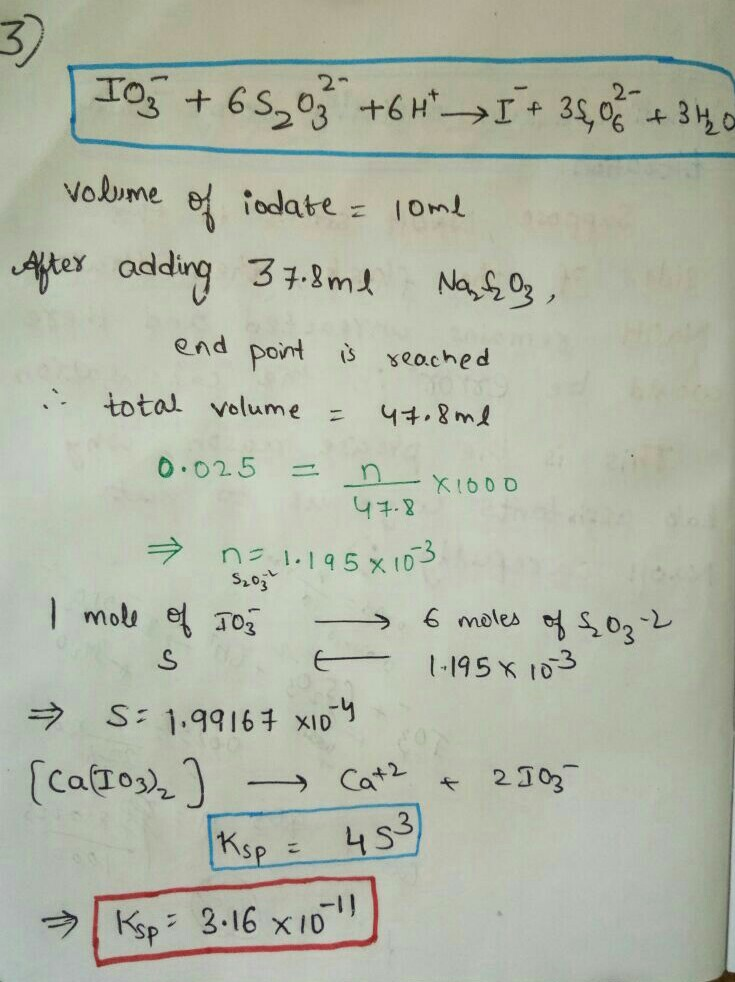# Question & Answer: In the Spontaneity experiment, the following data were collected by a student: The…..In the Spontaneity experiment, the following data were collected by a student: The mass of NaNO_3 taken was 8.5 g and the volume of water taken was 100 ml. The specific heat of water is 4.184 J and the heat capacity of the calorimeter is 41.84 J. Calculate the Heat of this reaction for 1 mol of NaNO_3. (Delta H) In an experiment to calculate the K_sp of Calcium Iodate [Ca(IO_3)_2], a 10 mL solution of a saturated solution of Calcium Iodate was titrated against 0.0250 M solution of Sodium thiosulfate (Na_2S_2O_3) using starch indicator. The end point of the titration was reached at 37.8 mL of Sodium thiosulfate. The reaction is, IO^-_3 + 6 S_2 O^2_3 + 6H^+ rightarrow I^- + 3 S_4O^2-_6 + 3H_2O Calculate the Solubility Product of Calcium Iodate.